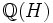# Perlis-Walker theorem

Let$G$ and$H$ be finite Abelian groups. Then, the group algebra$\mathbb{Q}(G)$ is isomorphic to the group algebra$\mathbb{Q}(H)$ if and only if$G$ is isomorphic to$H$.